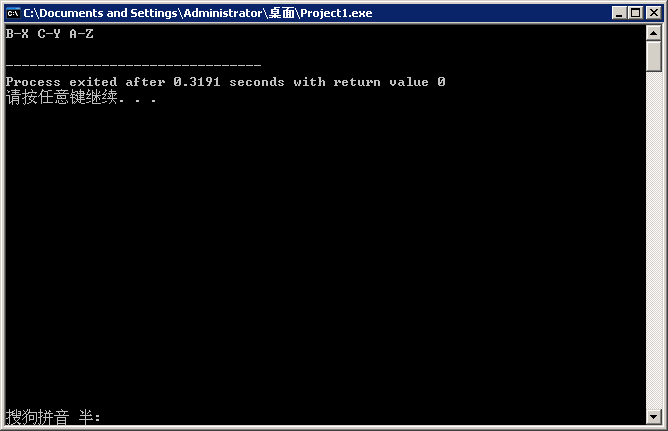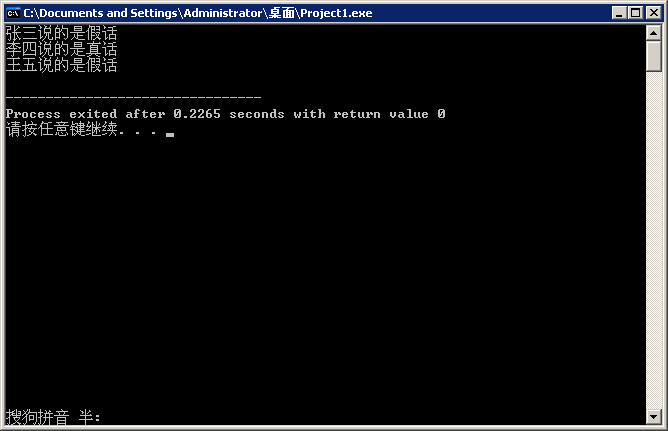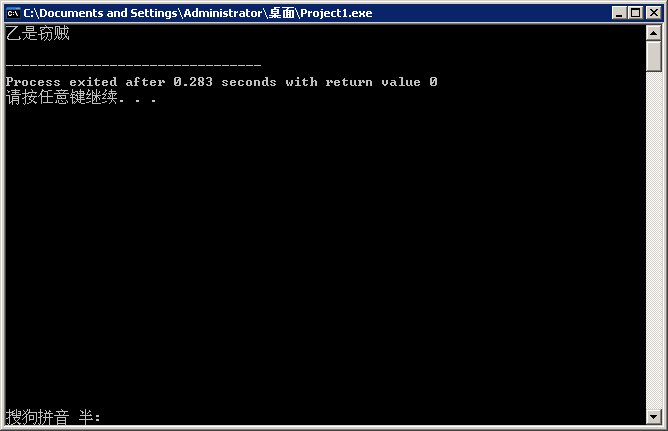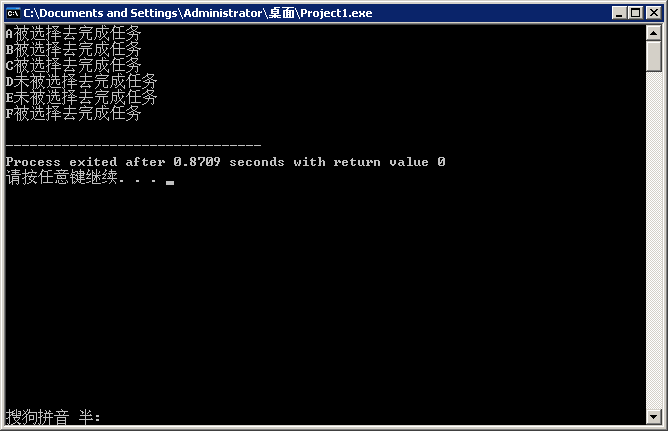蓝桥杯——趣味逻辑推理一（2017.2.16）

1. 新郎和新娘

三对情侣参加婚礼，三个新郞为A、B、C，三个新娘为X、Y、Z。有人不知道谁和谁结婚，于是询问了六位新人中的三位，但听到的回答是这样的：A说他将和X结婚；X说她的未婚夫是C；C说他将和Z结婚。这人听后知道他们在开玩笑，全是假话。请编程找出谁将和谁结婚。

【分析】可设三个新郎为x, y, z，穷举所有情况；

根据三句假话可推断：x!='A'  x!='C'  z!='C'，且根据ABC与XYZ的一一对应关系可知x!=y  y!=z  x!=z。这些条件需同时成立。

#include <stdio.h>
int main()
{
char x,y,z;                        //xyz分别为与新娘XYZ结婚的新郎
for(x='A';x<='C';x++)              //三重循环穷举所有可能情况
{
for(y='A';y<='C';y++)
{
for(z='A';z<='C' && x!=y;z++)
{
if(x!='A' && x!='C' && z!='C' && x!=z && y!=z)  //三句假话+一个新郎只能与一个新娘结婚
printf("%c-%c %c-%c %c-%c\n",x,'X',y,'Y',z,'Z');
}
}
}
return 0;
}2. 谁在说谎？

现有张三、李四和王五三个人：

张三说李四在说谎，

李四说王五在说谎，

而王五说张三和李四两人都在说谎，

要求编程求出这3个人中到底谁说的是真话，谁说的是假话。

【分析】

题中逻辑条件：由于不知道三个人说的真与假，故有以下几种可能情况：

1. 张三真李四假或 张三假李四真（互反）

2. 李四真王五假或 李四假王五真（互反）

3. 王五真、张三李四均假  或  王五假、张三李四一真一假

可设i, j, k分别为张三、李四、王五，真假分别用1和0表示，枚举所有可能情况。

#include <stdio.h>
int main()
{
int i,j,k;                 //i-张三 j-李四 k-王五
for(i=0;i<=1;i++)          //1真0假
{
for(j=0;j<=1;j++)
{
for(k=0;k<=1;k++)
{
if(((i&&!j)||(!i&&j)) && ((j&&!k)||(!j&&k)) && ((k&&!i&&!j) || !k&&(i+j!=0)))
{
printf("张三说的是%s\n",i?"真话":"假话");
printf("李四说的是%s\n",j?"真话":"假话");
printf("王五说的是%s\n",k?"真话":"假话");
}
}
}
}
return 0;
}3. 谁是窃贼

警察审问四名窃贼嫌疑犯。已知，这四人当中仅有一名是窃贼，还知道这四个人中每人要么是诚实的，要么总是说谎。他们给警察的回答是：
甲说：“乙没有偷，是丁偷的” ；
乙说：“我没有偷，是丙偷的” ；
丙说：“甲没有偷，是乙偷的”；
丁说：“我没有偷”。

请编写程序，根据这四个人的回答判断谁是窃贼。

【分析】可用变量ABCD分别代表甲乙丙丁四人，1和0分别代表该日是/不是窃贼，则可得出以下条件：

B+D=1，B+C=1，A+B=1，A+B+C+D=1；

由于甲乙丙三人的话中都提到了两个人，其中必有一人是小偷，所以在根据他们的话列出条件表达式时可不必关心谁说的是真话谁说的是假话（丁的话陈述了一个“客观条件”即四人中有仅有一名是窃贼，故无法判断真假）；

可依次设ABCD为窃贼，代入前三个表达式相与后的表达式依次检验。

#include <stdio.h>
int main()
{
int i;
int A=1,B=0,C=0,D=0;             //假定A为窃贼(1000)
for(i=1;i<=4;i++)
{
if(B+D==1 && B+C==1 && A+B==1)
break;
else
{
if(i==1)                 //甲不是窃贼，测试乙是否是窃贼(0100)
A=0,B=1;
if(i==2)                 //甲乙都不是窃贼，测试丙是否是窃贼(0010)
B=0,C=1;
if(i==3)                 //甲乙丙都不是窃贼，测试丁是否是窃贼(0001)
C=0,D=1;
}
}
if(i==1)
printf("甲是窃贼\n");
if(i==2)
printf("乙是窃贼\n");
if(i==3)
printf("丙是窃贼\n");
if(i==4)
printf("丁是窃贼\n");
return 0;
}4. 委派任务

某侦察队接到一项紧急任务，要求在A、B、C、D、E、F六个队员中尽可能多地挑若干人，但有以下限制条件：
（1）A和B两人中至少去一人；
（2）A和D不能一起去；
（3）A、E和F三人中要派两人去；
（4）B和C都去或都不去；
（5）C和D两人中只能去一个；
（6）若D不去，则E也不去。
问应当让哪几个人去？

【分析】可用ABCDEF 6个变量分别表示这6个人，某个人被选定去完成任务对应1，否则对应0，因此可得以下6个表达式：

A+B>=1，A+D!=2，A+E+F==2，B+C==0 || B+C==2（都去2，都不去0），C+D==1，D+E==0 || D==1（DE都不去 或 D去，E去不去均可）

联立求解即可。

#include <stdio.h>
int main()
{
int A,B,C,D,E,F;
for(A=0;A<=1;A++)
{
for(B=0;B<=1;B++)
{
for(C=0;C<=1;C++)
{
for(D=0;D<=1;D++)
{
for(E=0;E<=1;E++)
{
for(F=0;F<=1;F++)
{
if(A+B>=1 && A+D!=2 && A+E+F==2 && (B+C==0||B+C==2) && C+D==1 && (D+E==0||D==1))
{
printf("A%s被选择去完成任务\n",A?"":"未");
printf("B%s被选择去完成任务\n",B?"":"未");
printf("C%s被选择去完成任务\n",C?"":"未");
printf("D%s被选择去完成任务\n",D?"":"未");
printf("E%s被选择去完成任务\n",E?"":"未");
printf("F%s被选择去完成任务\n",F?"":"未");
}
}
}
}
}
}
}
return 0;
}